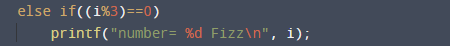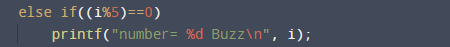Buzz Fizz Program in C

In this tutorial you will learn about the Buzz Fizz Program in C and its application with practical example.

In this tutorial, we will learn to create a C program that  Find BUZZ FIZZ  using C programming.

Prerequisites

Before starting with this tutorial we assume that you are best aware of the following C programming topics:

• Operators
• Basic C programming.
• Basic input/output.
• Looping statements
• For Loop
• If else statement.

What Is Buzz Fizz?

The concept of Buzz and Fizz is if integers between 1 to N, print “Fizz” if an integer is divisible by 3, “Buzz” if an integer is divisible by 5, and “FizzBuzz” if an integer is divisible by both 3 and 5.

Example:

A program that prints the numbers from 1 to no(suppose 15) .If number is a multiple of ‘3’ print “Fizz” instead if multiples of ‘5’ print “Buzz” and if multiple of 3 and 5 both then print “Fizz Buzz”.
1, 2, Fizz, 4, Buzz, Fizz, 7, 8, Fizz, Buzz, 11, Fizz, 13, 14,
Fizz Buzz.

Buzz Fizz Program in C.

In this program we will print find  Buzz Fizz  using for loop. We would first declared and initialized the required variables. Next, we would prompt user to input the number of terms. Later we will  find  Buzz Fizz series of specified number of terms.

OutputIn the above program, we have first declared and initialized a set variables required in the program.

• no = number of terms to be printed
• i     = for iteration of loop.

Here in this program we will print  number from 1 to no.If number is  multiple of 3, we print “Fizz” instead of the number. as you can see in image below.And if number is multiple of 5, print “Buzz” instead of the number.And in final case if numbers which are multiples of both 3 and 5, print “FizzBuzz” instead of the number.So in our program we take a number from user at run time,suppose user gives a number 15 then the series of fizz , buzz  and fizzbuzz is look like this.In this tutorial we have learn about the Buzz Fizz Program in C and its application with practical example. I hope you will like this tutorial.